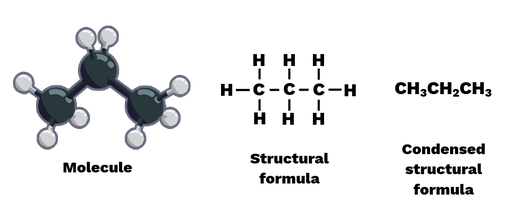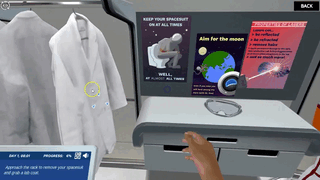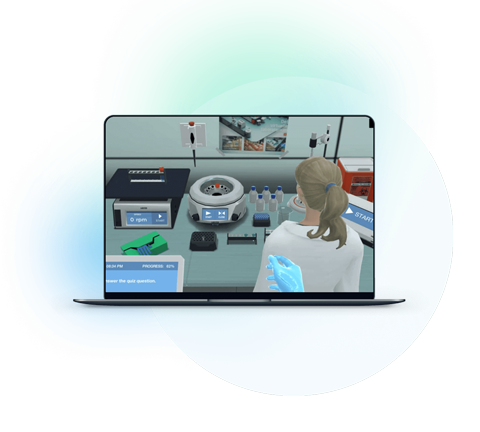Blog

# 5 Ways to Make Formulas and Equation Balancing Interesting to Students

Grace Chukwuekwu
Teaching with Labster
October 17, 2022

Compounds and molecules are made up of many atoms bonded together. The chemical formula describes the type and number of these atoms. For example, take the chemical formula for water: H2O. This formula shows that water consists of one oxygen atom (O) and two hydrogen atoms (H). The number of each element is given as a subscript after each element unless the number is 1 (for oxygen).

As you read, here are some questions you will find answers to:

1. How do teachers explain chemical processes and balance equations to young learners?

2. How do they make students comfortable with the idea that chemical reactions are part of our everyday lives?

3. What are the benefits of a virtual lab?

## What Is The Difficulty In Learning Formula And Equation Balancing?

There are three reasons why formulas and equations balancing can be difficult for even the most committed student.

### 1. It Feels abstract

Because the balancing of formulas and equations is done at the molecular level, you cannot see or feel them. Not being able to behold the process and not see its relevance to the real world can frustrate learning and make it tough for learners to stay motivated.

### 2. This is content heavy

There are three main types of chemical formulas that contain varying degrees of structural information:

1. Empirical formula

2. Molecular Formula

3. Structural formula

Empirical formula:

The molecular formula of a compound is the smallest whole number ratio of the atoms in that compound. In other words, the empirical formula only gives you the ratio between each type of element in the compound, not the actual number of atoms in each molecule. For example, the molecular formula for glucose is CH2O, which means there are twice as many hydrogen atoms as there are carbon or oxygen atoms.

Molecular Formula:

The molecular formula shows the total number of each atom present in a molecule. Thus, the molecular formula is an integer multiple of the corresponding empirical formula. For example, glucose has the molecular formula CH2O. The molecular formula of glucose is C6H12O6, which is a multiple of six applied to the empirical formula.

Structural formula:

The structural formula provides more information about the structure of the molecule. This is an extended molecular formula that provides information about the grouping and/or bonds between atoms. The structural formula for propane describes the bonds between carbon and hydrogen atoms with lines. The abbreviated structural formula gives almost the same information due to the arrangement of the atoms.Figure: The molecular structure of propane can be illustrated by its structural formula and abbreviated structural formula.

### 3. It's complicated

A chemical reaction equation defines how one or more substances change their chemical composition to create new ones. Although new substances are formed, atoms are not destroyed or created. An example of a chemical reaction equation is

2H2 + O2 → 2H2O.

This equation depicts the reaction between hydrogen and oxygen to form water. Hydrogen and oxygen are reactants whereas water is a product of this reaction.

## 5 Ways To Improve The Understanding Of Formulas And Equation Balancing

With those points in mind, here are things you can incorporate into formulas and equations balancing lessons to make them more engaging, accessible, and fun for you and your students.

### 1. Show the people behind the science

Modern chemical nomenclature begins with Berzeluis, one of the so-called fathers of chemistry, when he said that chemicals should be given a name based on what they are and not where they came from. (After all, malic acid was found elsewhere, such as in cherries.) He created the 1- and 2-letter atomic symbol system taught in high school today, with letters taken from the Latin word for element (hence "Pb'' for plumbum which is Lead). This method of writing chemical formulas was developed by the 19th century Swedish chemist Jons Jacob Berzelius.

### 2. Relate to the real world

Coefficients and subscripts:

There are two types of numbers in a chemical equation: coefficients, which indicate the total number of molecules/atoms in a reaction, and subscripts, which indicate the amount of each element in the molecule. So 2H2O means we have two water molecules, each with two hydrogen atoms and one oxygen atom. This gives a total of four hydrogen atoms and two oxygen atoms.

### 3. Seeing is believing

Visualization can make all the difference when a topic is as complex and abstract as formulas and equation balancing.

Conservation of Mass:

When reactants react to form products, atoms are neither created nor destroyed. This principle is known as the law of conservation of mass. As a result, all chemical equations must be balanced. In a balanced reaction equation, there is an equal number of atoms for each element in the equation. For the reaction

2H2 + O2 → 2H2O,

The coefficients are added in front of the H2 and H2O molecules to balance the equation. Combined with the index, this coefficient gives four hydrogen atoms and two oxygen atoms on the reactant and product sides of the corresponding equation. A common example is when wood burns, the amount of soot, ash, and gas is equal to the initial charcoal and oxygen when it first reacted. Therefore, the mass of the product is equal to that of the reactant.

### 4. Make it stick with word-play

Visualization can assist in comprehending this lesson but when learning chemical reactions, chemical formulas, and equation balancing, there are few options other than the use of memory aids. An example is three types of chemical formulas that can be recalled as StEM where the lowercase alphabet is not considered as shown;

S - Structural formula

M - Molecular Formula

E - Empirical formula

### 5. Use virtual laboratory simulation

A unique way to teach formulas and equation balancing is through virtual laboratory simulations. At Labster, we strive to provide highly interactive lab presentations using game-based elements such as storytelling and scoring systems in an immersive 3D world.

Check out Labster formulas and equation balancing Simulation. You will learn the difference between reactants and products and how the conservation of mass law guides us and how to balance equations.